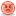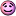CodeGuru Home VC++ / MFC / C++ .NET / C# Visual Basic VB Forums Developer.com

1.Junior MemberJoin Date
May 2010
Posts
4

##prim's algorithm

i want to write program for prim's algorithms in VB6 but i can't becouse i am new in VB6
and they want me to do it in one week
so help me if you canReply With Quote

2. ## Re: prim's algorithm

Not very likely you would get any help this way...

Regards,
ZachmReply With Quote

3.Junior MemberJoin Date
May 2010
Posts
4

## Re: prim's algorithm

thank you
i didnot knowReply With Quote

4.Junior MemberJoin Date
May 2010
Posts
4

## Re: prim's algorithm

but sir Zachm i want to tell some thing
i didn't study this subject and i dont know what is an algorithms
it just my supervisor tell me to do some thing new and i reject his idea in the biging but he tell me that i have to do it in VB6. it is easy.
then i try to under stand it until i get it in c++(i have the code for it) but i cant do it in Vb6 because i am studying the principle of it now
so help me if you can please by do it in VB6 or convert the code of c++ and this is the code in c++ :

#include<iostream.h>
class prims
{
private:
int n; //no of nodes
int graph_edge; //edges in the graph
int g; //no of edges in the graph
int tree_edge; //edges in the tree
int t; //no of edges in the tree
int s; //source node
//Partition the graph in to two sets
int T1,t1; // Set 1
int T2,t2; // Set 2
public:
void input();
int findset(int);
void algorithm();
void output();
};
void prims::input()
{
cout<<”*************************************************\n”
<<”This program implements the prims algorithm\n”
<<”*************************************************\n”;
cout<<”Enter the no. of nodes in the undirected weighted graph ::”;
cin>>n;
g=0;
cout<<”Enter the weights for the following edges ::\n”;
for(int i=1;i<=n;i++)
{
for(int j=i+1;j<=n;j++)
{
cout<<” < “<<i<<” , “<<j<<” > ::”;
int w;
cin>>w;
if(w!=0)
{
g++;
graph_edge[g]=i;
graph_edge[g]=j;
graph_edge[g]=w;
}
}
}
// print the graph edges
cout<<”\n\nThe edges in the given graph are::\n”;
for(i=1;i<=g;i++)
cout<<” < “<<graph_edge[i]
<<” , “<<graph_edge[i]
<<” > ::”<<graph_edge[i]<<endl;
}
int prims::findset(int x)
{
for(int i=1;i<=t1;i++)
if(x==T1[i])
return 1;
for(i=1;i<=t2;i++)
if(x==T2[i])
return 2;
return -1;
}
void prims::algorithm()
{
t=0;
t1=1;
T1=1; //The source node
t2=n-1;
int i;
for(i=1;i<=n-1;i++)
T2[i]=i+1; //The reamining nodes
cout<<”\n*****The algorithm starts*****\n\n”;
while(g!=0 && t!=n-1)
{
// Find the least cost edge
int min=9999;
int p;
int u,v,w;
for(i=1;i<=g;i++)
{
bool flag1=false,flag2=false;
//if u and v are in different sets
if(findset(graph_edge[i])!=findset(graph_edge[i]))
{
if(min>graph_edge[i])
{
min=graph_edge[i];
u=graph_edge[i];
v=graph_edge[i];
w=graph_edge[i];
p=i;
}
}
}
//break if there is no such edge
cout<<”The edge included in the tree is ::”;
cout<<” < “<<u<<” , “<<v<<” > “<<endl;
//delete the edge from graph edges
for(int l=p;l<g;l++)
{
graph_edge[l]=graph_edge[l+1];
graph_edge[l]=graph_edge[l+1];
graph_edge[l]=graph_edge[l+1];
}
g–;
//add the edge to the tree
t++;
tree_edge[t]=u;
tree_edge[t]=v;
tree_edge[t]=w;
//Alter the set partitions
t1++;
int m;
if(findset(v)==2)
{
T1[t1]=v;
m=v;
}
else if(findset(u)==2)
{
T1[t1]=u;
m=u;
}
int x;
for(x=1;T2[x]!=m;x++);
for(;x<t2;x++)
T2[x]=T2[x+1];
t2–;
// Print the sets
int k;
cout<<”NOW\nT1 :: “;
for(k=1;k<=t1;k++)
cout<<T1[k]<<’ ‘;
cout<<endl;
cout<<”T2 :: “;
for(k=1;k<=t2;k++)
cout<<T2[k]<<’ ‘;
cout<<endl;
cout<<”The graph edges are ::\n”;
for(i=1;i<=g;i++)
cout<<” < “<<graph_edge[i]
<<” , “<<graph_edge[i]
<<” > ::”<<graph_edge[i]<<endl;
cout<<endl<<endl;
}
}
void prims:utput()
{
cout<<”\nThe selected edges are ::\n”;
for(int i=1;i<=t;i++)
cout<<” < “<<tree_edge[i]
<<” , “<<tree_edge[i]
<<” > ::”<<tree_edge[i]<<endl;
}
int main()
{
prims obj;
obj.input();
obj.algorithm();
obj.output();
return 0;
}Reply With Quote

5. ## Re: prim's algorithm

I don't think you got the point.
The whole point of homework is that you will learn something new by thinking, experiencing and studying yourself. If someone here did it for you, then you would miss the point of homework and of these forums.

If your supervisor makes demands that you think are unreasonable, this is no one's fault but your supervisor's, or yourself, if the demands turn out to be reasonable, so please don't throw the mercy card at us (plus, it looks bad for you).

People here will be totally willing to help you with your homework, once you at least show some effort, post some code you are having difficulty with, etc.

I am not trying to attack you, or scare you away, just point out that no one here is likely to do the whole work for you, even if they can, for the specified reasons.

This forum is for algorithms, so once you make some effort, or at least ask specific questions, you should probably post in the VB forum.

Regards,
ZachmReply With Quote

6.Junior MemberJoin Date
May 2010
Posts
4

## Re: prim's algorithm

thank you againReply With Quote

####Posting Permissions

• You may not post new threads
• You may not post replies
• You may not post attachments
• You may not edit your posts
•